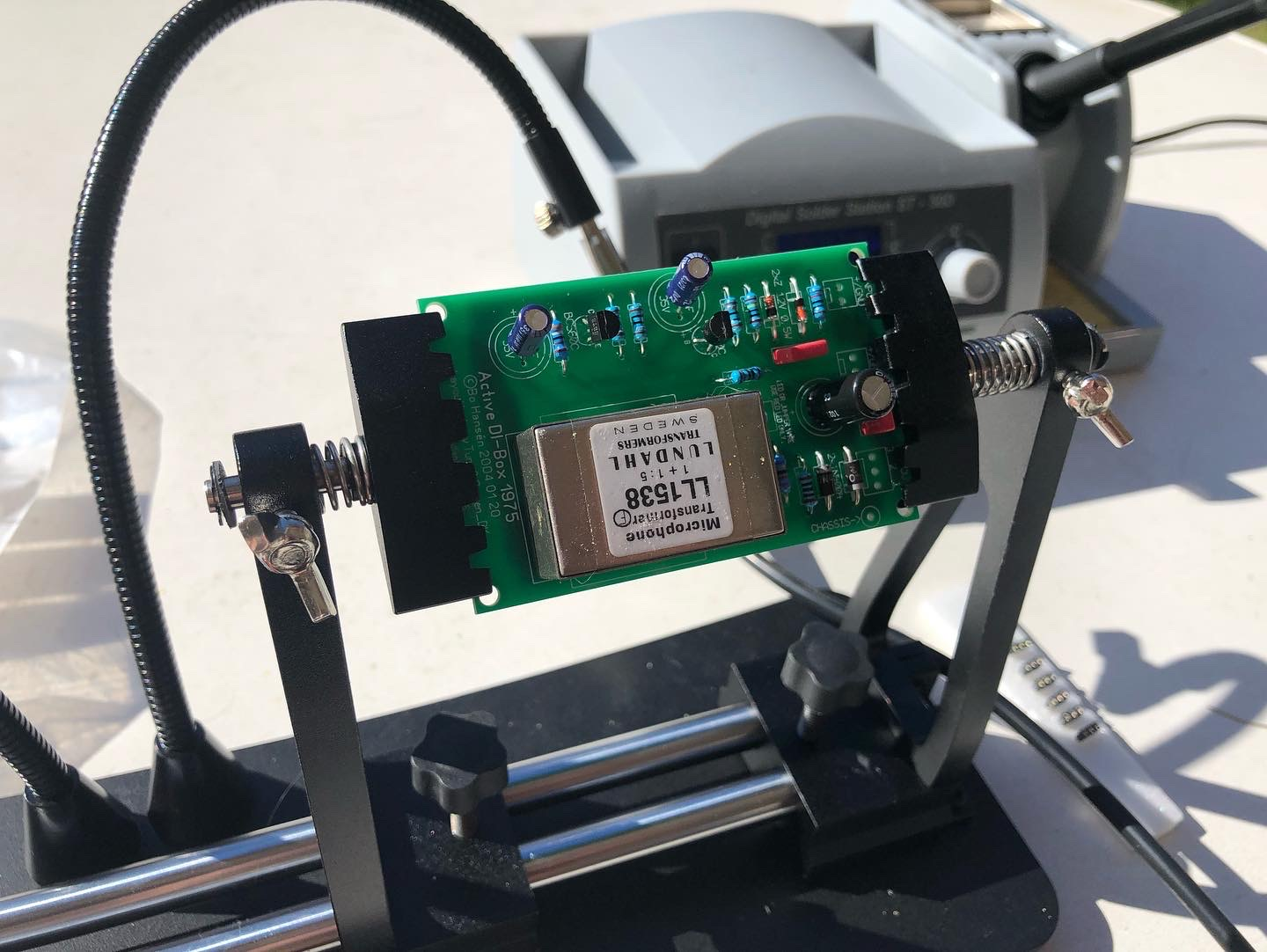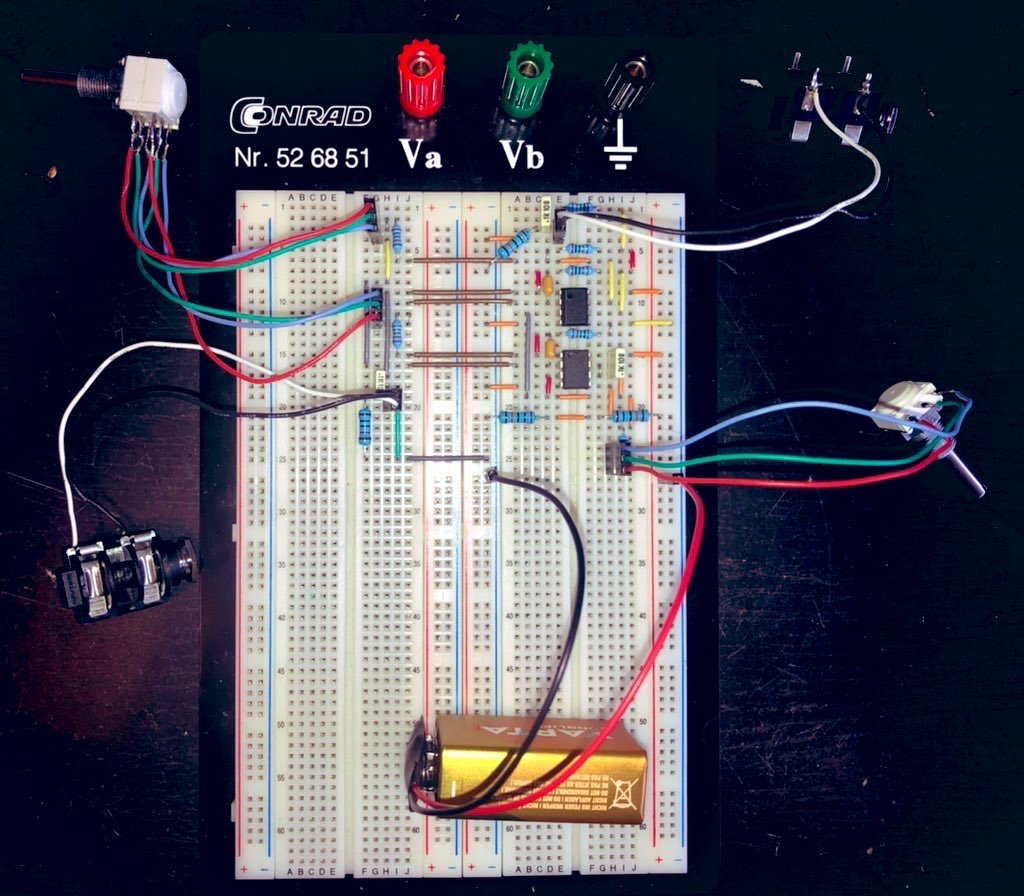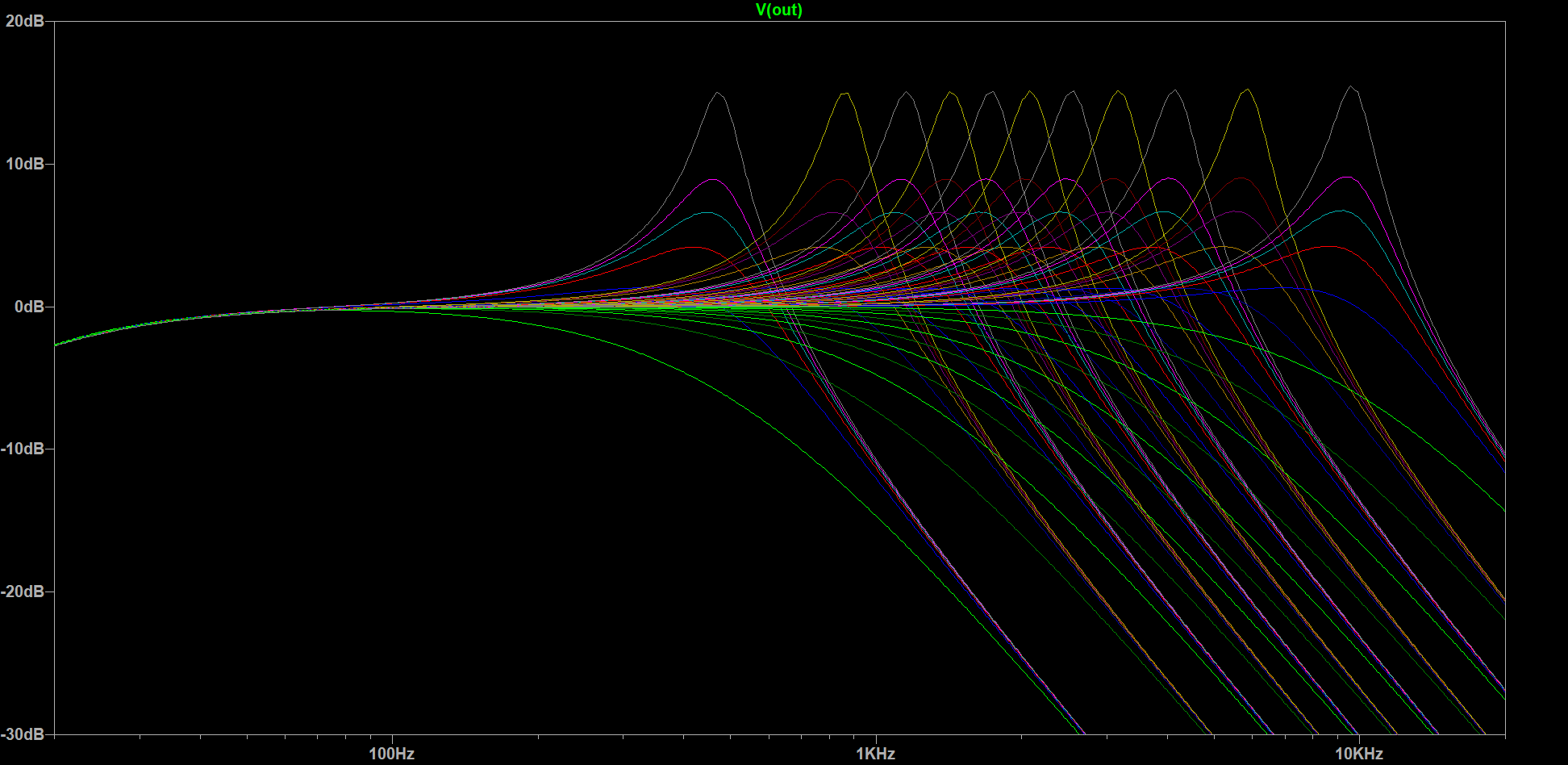## A Better SVF Preamp

In an­oth­er di­gres­sion from my run­ning se­ries of posts on us­ing Rust to pro­gram the Bela, I de­cid­ed to do some ana­log au­dio pro­cess­ing. Why ana­log in­stead of the more fa­mil­iar to me dig­i­tal sig­nal pro­cess­ing route? There are sev­er­al rea­sons, one even re­lat­ed to the afore­men­tioned se­ries!

First, the un­re­lat­ed rea­son: I was sort­ing through some old box­es while look­ing for some­thing and dis­cov­ered my long-un­used col­lec­tion of elec­tron­ics com­po­nents. In­clud­ing a set of com­po­nents that looked a lot like some kind of au­dio project, for­got­ten for more than a decade—yes, re­al­ly, the ship­ping dates were on the la­bels! The (ex­pen­sive) Lun­dahl LL1538 au­dio trans­former was a good hint, but the slight­ly yel­lowed schemat­ic for a Bo Han­sén 1975 DI made it clear what it was. So I or­dered a PCB from 51x­Au­dio and the hand­ful of miss­ing—prob­a­bly lost in trans­port over the course of two moves—com­po­nents and fi­nal­ly fin­ished the project:And it worked im­me­di­ate­ly! With the elec­tron­ics bug in me re­vived (the “en­thu­si­asm”-kind not the “pro­gram­ming er­ror”-kind), I got back to think­ing about the next project I plan with the Bela—which brings us to the sec­ond, Bela-re­lat­ed rea­son why I'm do­ing this. Yeah, yeah, “fin­ish the first one first,” I hear you say, but I an­nounced this one right in the sec­ond post al­ready! If you don't re­mem­ber and aren't in the mood to go back to the pre­vi­ous post, here's the TL;DR: I plan to build a poly­phon­ic (bass) gui­tar us­ing Cy­c­fi Nu mod­u­lar pick­ups, us­ing the Bela to per­form per-string au­dio pro­cess­ing.

But wait, isn't that just more dig­i­tal pro­cess­ing? It is, but a poly­phon­ic set­up is a com­plex beast, typ­i­cal­ly re­quir­ing ad­di­tion­al con­trollers, mul­ti­ple am­pli­fiers, etc.; and some­times you just want to plug and play. And to do that, you re­al­ly want some­thing that be­haves like an or­di­nary, mono­phon­ic pick­up, which the Nus are not, in mul­ti­ple ways:

1. They are ac­tive, i.e., they re­quire a bat­tery.
2. They are poly­phon­ic (duh) and there­fore out­put per-string sig­nals in­stead of a sin­gle sig­nal for all strings.
3. In­ter­nal­ly, the cap­sules use low-Z, wide-band­width pick­ups.

The first dif­fer­ence isn't much of an is­sue, and is par for the course for bass gui­tars, where ac­tive pick­ups and/or ac­tive preamps are very com­mon—two of the three I own have a bat­tery com­part­ment. Gui­tar play­ers are of­ten a lit­tle more con­ser­va­tive, but even there, ac­tive pick­ups, such as EMGs or Fish­mans, are pop­u­lar in some gen­res.

The sec­ond dif­fer­ence is eas­i­ly re­solved us­ing a sum­ming am­pli­fi­er. A sum­ming am­pli­fi­er is the ba­sis of any ac­tive mix­er and con­sists of a sin­gle op-amp (op­er­a­tional am­pli­fi­er) and a hand­ful of re­sis­tors—and in most cas­es some de­cou­pling ca­pac­i­tors to stop any DC off­sets from prop­a­gat­ing. If you are in­ter­est­ed in an over­view of how sum­ming am­pli­fiers work and why they are typ­i­cal­ly bet­ter than pas­sive mix­ers—such as the av­er­age mul­ti-pick­up bass's bal­ance con­trol—check out El­liot Sound Prod­uct's ar­ti­cle on au­dio sig­nal mix­ing. They gen­er­al­ly have a lot of great ar­ti­cles on au­dio elec­tron­ics, so def­i­nite­ly worth check­ing out if you are in­ter­est­ed in these things—and I as­sume you are, hav­ing read this far.

The third is­sue is where things get more com­pli­cat­ed. First off, what does low-Z and wide band­width even mean in this con­text? Low-Z is short for low im­ped­ance (Z be­ing the stan­dard sym­bol used for im­ped­ances in elec­tron­ics lit­er­a­ture). Es­sen­tial­ly, im­ped­ance is the fre­quen­cy-de­pen­dent ana­log to a re­sis­tance for AC sig­nals. Typ­i­cal­ly, a low-Z pick­up will have few­er wind­ings and there­fore low­er DC re­sis­tance, low­er in­duc­tance, and low­er wind­ing ca­pac­i­tance than a reg­u­lar pick­up. This leads to a wider band­width, i.e., they pick up high­er fre­quen­cies, typ­i­cal­ly with­out los­ing low-fre­quen­cy re­sponse, and a res­o­nance fre­quen­cy out­side the au­di­ble fre­quen­cy spec­trum; but al­so sig­nif­i­cant­ly re­duced out­put, lead­ing to the ne­ces­si­ty of ac­tive elec­tron­ics (or trans­form­ers in Lace Alu­mi­tones).

Wait. Res­o­nance fre­quen­cy? Yes, all coils, in­clud­ing trans­form­ers and pick­ups, have a res­o­nance fre­quen­cy, de­ter­mined by their in­duc­tance and wind­ing ca­pac­i­tance—as well as any ex­ter­nal loads, such as long ca­bles or a rolled down tone knob. These form a res­o­nant, sec­ond-or­der (12 dB/oc­tave) low-pass fil­ter. The oth­er par­a­sitics of the coil af­fect the so-called Q of this fil­ter. Q is of­ten de­scribed as band­width, but that on­ly re­al­ly ap­plies to sec­ond-or­der band-pass fil­ters. For low-pass fil­ters it makes more sense to think about Q as the gain at the res­o­nant fre­quen­cy.

Is this res­o­nance de­sir­able? Well, yes and no. In au­dio trans­form­ers for pro­fes­sion­al au­dio ap­pli­ca­tions, it is un­de­sir­able and a Zo­bel net­work is used to tame the res­o­nance and en­sure a flat re­sponse across the spec­trum (check out ESP for de­tails). Gui­tar pick­ups are a dif­fer­ent sto­ry. Here, the res­o­nance is usu­al­ly smack dab in the mid­dle of the au­dio spec­trum—with res­o­nance fre­quen­cies rang­ing from 800 Hz for hum­buck­ers to 8 kHz for sin­gle coils—and a large fac­tor in the per­ceived “tone” of the pick­up. In fact, an am­pli­fi­er with a low in­put im­ped­ance will lead to sig­nif­i­cant damp­ing of the res­o­nance lead­ing to a tone that is of­ten de­scribed as “dead.” By the way, a DI box as shown above is used con­vert a high-im­ped­ance sig­nal to a bal­anced low-im­ped­ance sig­nal for a mi­cro­phone preamp when record­ing. In any case, gi­tar­renelek­tron­ik.de has a num­ber of good ar­ti­cles on gui­tar pick­up res­o­nance and how to af­fect it (al­beit on­ly in Ger­man).

So, how can we em­u­late the re­sponse of clas­sic pas­sive pick­up with a wide band­width ac­tive pick­up? There are a cou­ple op­tions. One op­tion would be to add a pas­sive RLC-net­work, op­tion­al­ly with switch­able ca­pac­i­tors and re­sis­tors, al­though that would be af­fect­ed by ex­ter­nal loads too, un­less an ac­tive buf­fer is added. An­oth­er op­tion, the route I chose, is to use an ac­tive fil­ter. A great fil­ter topol­o­gy for this ef­fect is the state vari­able fil­ter:While oth­er sec­ond-or­der fil­ter topolo­gies re­quire few­er than three op-amps, the SVF topol­o­gy al­lows in­de­pen­dent con­trol of fre­quen­cy and Q. Ad­di­tion­al­ly, both Alem­bic and Wal, two of the most pre­mi­um/lux­u­ry-lev­el bass builders al­ready use ac­tive low-pass fil­ters in their preamps! Pre­sum­ably, they use SVFs, al­though it isn't re­al­ly doc­u­ment­ed, since their elec­tron­ics are pro­pri­etary. For an over­view of ac­tive fil­ters for au­dio, you can, again, check out ESP.

Now let's look in­to the cir­cuit in more de­tail! In the schemat­ic above, fre­quen­cy and Q are set by

$f = \frac{1}{2 \pi R_F C}$

and

$Q = \frac{1}{3 g},$

where $g$ is the volt­age di­vider gain

$g = \frac{R_{Q2}}{R_{Q1} + R_{Q2}}.$

In the lit­er­a­ture, vari­able SVFs are typ­i­cal­ly di­men­sioned by re­plac­ing the re­sis­tors $R_F$ with a du­al-gang rheo­stat (vari­able re­sis­tor) ad­justable be­tween 0 Ω and $\mathit{RV}_F$ in se­ries with fixed re­sis­tors $R_{F0}$ each. Along with the ca­pac­i­tors $C$ this sets the fre­quen­cy range to

$f_\mathrm{min} = \frac{1}{2 \pi (\mathit{RV}_F + R_{F0}) C}$ $f_\mathrm{max} = \frac{1}{2 \pi R_{F0} C}$

Sim­i­lar­ly, Q can be con­trolled by re­plac­ing ei­ther $R_{Q1}$ or $R_{Q2}$ by a rheo­stat $\mathit{RV}_Q$ in se­ries with a fixed re­sis­tor $R_{Q0}$ . If $R_{Q1}$ is kept fixed and $R_{Q2}$ is var­ied, this re­sults in a Q be­tween

$Q_\mathrm{min} = \frac{1 + \frac{R_{Q1}}{\mathit{RV}_Q + R_{Q0}}}{3}$

and

$Q_\mathrm{max} = \frac{1 + \frac{R_{Q1}}{R_{Q0}}}{3}.$

I plugged in the num­bers for my de­sired fre­quen­cy ad­just­ment range of 500 Hz to 10 kHz and Q ad­just­ment range of 0.5 (-6.02 dB) to 5.62 (+15 dB), and the 50 kΩ of the po­ten­tiome­ters—vari­able volt­age di­viders that can be wired as rheostats by short­ing the wiper to one of the oth­er two pins—re­sult­ing in $R_{F0} \approx 2.61\mathrm{k\Omega}$ , $C \approx 6.2\mathrm{nF}$ , $R_{Q0} \approx 1.62\mathrm{k\Omega}$ , and $R_{Q1} \approx 25.5\mathrm{k\Omega}$ . Then I got all the nec­es­sary parts and set them up on a bread­board.And it worked. Kind of. While it did what it was sup­posed to, the ad­just­ment range of the po­ten­tiome­ters was down­right ter­ri­ble, with more than 80% of the ad­just­ment hap­pen­ing in the last 20% or so of the ro­ta­tion range. Not ex­act­ly us­able on stage. One method to solve this is to use po­ten­tiome­ters with a dif­fer­ent ta­per. In this case, due to the re­cip­ro­cal na­ture of our ranges, it would have to be a re­verse au­dio/in­verse log­a­rith­mic ta­per. Hard to source, es­pe­cial­ly as an in­di­vid­u­al, and the con­for­mance—how close the re­sis­tances of a du­al-gang po­ten­tiome­ter are to one an­oth­er—is gen­er­al­ly much worse than for lin­ear po­ten­tiome­ters. And you want them to be close, oth­er­wise the Q will fluc­tu­ate while ad­just­ing the fre­quen­cy.

Luck­i­ly, I was re­cent­ly in con­tact with Joel de Guz­man, prin­ci­pal ar­chi­tect and en­gi­neer at Cy­c­fi Re­search who is de­sign­ing a cus­tom Nu Mul­ti for me. And he al­so hap­pens to be a C++ de­vel­op­er like I am—Rust 🦀 is still most­ly re­strict­ed to hob­by work. He sug­gest­ed us­ing the po­ten­tiome­ters as ac­tu­al three-ter­mi­nal po­ten­tiome­ters in­stead of two-ter­mi­nal rheostats. So how does that work? Let's fig­ure it out!

To do that, we'll have a look at each of the fil­ter's stages in­di­vid­u­al­ly. So let's be­gin with the in­put / high-pass stage. Here, both the in­put and the out­puts of the oth­er two stages come to­geth­er as feed­back:So can we just use our po­ten­tiome­ter to com­plete­ly re­place $R_{Q1}$ and $R_{Q2}$ , since they al­ready form a volt­age di­vider? Well, not re­al­ly. While the one end of the ad­just­ment range would re­sult in a Q of ⅓ (-9.54 dB), which is far too high­ly damped to be us­able but could po­ten­tial­ly work, the oth­er end of the range would re­sult in a Q of… ∞. Not good. So we def­i­nite­ly need re­sis­tors at both ends of the po­ten­tiome­ter. But just adding those would re­sult in a sim­i­lar­ly ill-suit­ed ad­just­ment range as be­fore, so what can we do? We can fake a non-lin­ear ta­per by adding a “shap­ing” re­sis­tor in par­al­lel to one of the sides, i.e., be­tween the wiper and one of the two ter­mi­nals, of the po­ten­tiome­ter as de­scribed in an old Ge­oFex ar­ti­cle. In this case, be­tween the wiper and ter­mi­nal three (the clock­wise ter­mi­nal) of the po­ten­tiome­ter:To sim­pli­fy the cal­cu­la­tions, let's use re­sis­tances rel­a­tive to $\mathit{RV}_Q$ , the to­tal re­sis­tance of the po­ten­tiome­ter, des­ig­nat­ed by low­er­case $r$ . This re­sults in

$g = \frac{r_{Q\mathrm{cw}} + ((1-t) \parallel r_{QS})}{r_{Q\mathrm{cw}} + ((1-t) \parallel r_{QS}) + t + r_{Q\mathrm{ccw}}},$

where $t \in [0, 1]$ is the trav­el of the po­ten­tiome­ter. By plug­ging in $t = 0$ and $Q_\mathrm{min}$ as well as $t = 1$ and $Q_\mathrm{max}$ in­to our volt­age di­vider and Q-equa­tion, we ar­rive at

$r_{Q\mathrm{cw}} = \frac{\frac{3 Q_\mathrm{min} - 1}{1 + \frac{1}{r_{QS}}} + 1}{3 (Q_\mathrm{max} - Q_\mathrm{min})}$

and

$r_{Q\mathrm{ccw}} = (3 Q_\mathrm{max} - 1) r_{Q\mathrm{cw}} - 1.$

How do we choose $r_{QS}$ ? I just put tried to plug in a few val­ues, and the op­ti­mum ap­pears to be some­where be­tween $\frac{1}{14}$ and $\frac{1}{12}$ for my de­sired ad­just­ment range. For oth­er ranges it will be dif­fer­ent. If you felt like it, you could even run an op­ti­miz­er to find the low­est pos­si­ble er­ror across the en­tire range. But with $R_{QS} = 3571\mathrm{\Omega}$ , $R_{Q\mathrm{cw}} = 3361\mathrm{\Omega}$ , and $R_{Q\mathrm{ccw}} = 3347\mathrm{\Omega}$ for $\mathit{RV}_Q = 50\mathrm{k\Omega}$ . Ob­vi­ous­ly, we still have to round these to ac­tu­al­ly avail­able E se­ries val­ues, but they re­sult in very good ad­just­ment be­hav­ior:The fre­quen­cy is con­trolled by the two es­sen­tial­ly iden­ti­cal in­te­gra­tor stages. So let's just look at one of them:What $R_F$ does here is a lit­tle less ob­vi­ous. In the pre­vi­ous case, we had a volt­age di­vider con­nect­ed to the high-im­ped­ance non-in­vert­ing in­put of an op-amp. Here, what we have is just a sin­gle re­sis­tor con­nect­ed to the in­vert­ing in­put, which I la­beled as vir­tu­al ground. What does that mean? Well, op-amps have a very high gain and the neg­a­tive feed­back will try to push the node at the in­vert­ing in­put to as close as the same volt­age as is present at the non-in­vert­ing in­put of the op-amp. Which in this case is ground. So in­stead of a very high-im­ped­ance volt­age in­put, we es­sen­tial­ly have a very low-im­ped­ance cur­rent in­put and $R_F$ con­trols the cur­rent flow­ing in­to the node at the in­vert­ing in­put! So what can we do with a po­ten­tiome­ter in this po­si­tion? We can wire it up as a “cur­rent split­ter” that con­trols both how much cur­rent flows from the pre­vi­ous stages out­put and how much of it flows to ground or to vir­tu­al ground:Here $i_0$ is the cur­rent flow­ing out of the pre­vi­ous stage's out­put and $i_\mathrm{in}$ is the cur­rent flow­ing in­to the vir­tu­al ground node. To sim­pli­fy cal­cu­la­tions, let's de­scribe the above cir­cuit us­ing fixed re­sis­tors:Since both the in­vert­ing in­put and ground are at 0 V, $R$ and $R_b$ are es­sen­tial­ly par­al­lel re­sis­tors for the pur­pose of com­put­ing $i_0$ :

$i_0 = \frac{u_\mathrm{prev}}{R_a + (R_b \parallel R)}$

$i_\mathrm{in}$ can now be com­put­ed us­ing stan­dard cur­rent di­vider rules:

\begin{aligned} i_\mathrm{in} &= i_0 \frac{R_b \parallel R}{R} \\ &= \frac{u_\mathrm{prev}}{\left(\frac{R_a}{R_b} + 1\right)R + R_a} \end{aligned}

Ef­fec­tive­ly, at least for the pur­pose of com­put­ing $i_\mathrm{in}$ , our cur­rent split­ter acts like a re­sis­tor

$R_F \equiv \left(\frac{R_a}{R_b} + 1\right)R + R_a,$

or in terms of re­sis­tances rel­a­tive to $\mathit{RV}_F$

$r_F \equiv \left(\frac{1-t}{t + r_{F\mathrm{ccw}}} + 1\right)r_{FS} + 1-t.$

As be­fore, we can plug in $t = 0$ and $t = 1$ and ar­bi­trar­i­ly choose $r_{FS}$ to op­ti­mize the ro­ta­tion­al be­hav­ior of our con­trol. $r_{F\mathrm{ccw}}$ is then de­ter­mined by

$r_{F\mathrm{ccw}} = \frac{1}{\frac{f_\mathrm{max}}{f_\mathrm{min}} - 1 - \frac{1}{r_{FS}}}$

For my de­sired fre­quen­cy range of 500 Hz to 10 kHz, $r_{FS} = \frac{2}{9}$ re­sults in a very good per­for­mance. Us­ing that val­ue and $\mathit{RV}_F = 50\mathrm{k\Omega}$ re­sults in $R_{FS} = 9091\mathrm{\Omega}$ , $R_{F\mathrm{ccw}} = 3704\mathrm{\Omega}$ , and $C = 1.8\mathrm{nF}$ . These re­sult in the fol­low­ing ad­just­ment curve:Are we done? Well, for this ar­ti­cle, pret­ty much, as build­ing the fil­ter is still a to-do. How­ev­er, I did plug it all in­to LT­Spice and sim­u­lat­ed it with re­al op-amp mod­els and an op-amp volt­age fol­low­er driv­en ground po­ten­tial. The lat­ter is nec­es­sary—and in­clud­ed in the bread­board cir­cuit above as well—since a bat­tery on­ly pro­vides a sin­gle volt­age, not a pos­i­tive and a neg­a­tive volt­age, so we have to fake it. In any case this is the re­sult­ing small-sig­nal gain:So the sim­u­la­tion agrees with all those cal­cu­la­tions, yay! Re­al­i­ty may turn out to be a lit­tle more com­plex, but I need to wait for some parts be­fore I can try it in prac­tice. Ad­di­tion­al­ly, the mod­i­fied cir­cuit in­cludes some fair­ly low im­ped­ances, which is good for noise per­for­mance, but bad for bat­tery life, so I may have to move to high­er-val­ue po­ten­tiome­ters as well—which have be­come rar­er as well for some rea­son. Maybe some­thing in the 200 to 500 kΩ range? Once I have bread­board­ed ev­ery­thing and de­cid­ed on com­po­nent val­ues, I'll ei­ther have to con­vert it to a perf­board lay­out or try to de­sign a PCB with Ki­CAD—more ex­pen­sive but far more com­pact, es­pe­cial­ly with SMD com­po­nents. In any case, that's it for to­day, and as usu­al, feel free to fol­low me and send me a DM on Twit­ter if you have any ques­tions or com­ments.